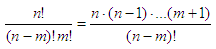# Factorials – several ideas to code them

### Four methods to code and calculate factorials

In mathematics, factorials of positive integers n, are the product of all positive integers less than or equal to n. Factorials are denoted by n!.

For example:
6! = 6 x 5 x 4 x 3 x 2 x 1 = 720

Note: 0! is a special case that is defined as 1.

The factorial operation is found in many areas of math, mainly in probability and statistics, combinatorics, algebra and data analysis. Its most basic appearance is due to the fact that there are n! ways to arrange n distinct elements into a sequence (permutations of any set of objects).

There are a number of solutions when we have to code factorials and we can experiment with any number-crunching software.

### 1. Function ‘factorial’

In Matlab, we can use built-in function ‘factorial’, like this:

n = 9
% use function factorial
factorial(n)

The answer is 362880.

### 2. Function ‘prod’

We can also use built-in function ‘prod’. For vectors, prod(n) is the product of the elements of n. For matrices, prod(X) is a row vector with the product of each column.

n = 9
% use function prod, which multiplicates
% every element in a vector

prod([1 : n])

The answer is, again, 362880.

### 3. Iterations

We can use iterations to get the multiplication of numbers, just following the definition of what a factorial is

n = 9
% use iterations
f = 1;
for i = 1:n
f = f*i;

end
f

### 4. Recursion

We could even use a recursive method to calculate a factorial. That idea and method is explained in detail in the article recursion.

### Custom-made formula

Now, if we want to calculate a custom-made formula including factorial operations, we can create and optimize a function for that specific purpose. Let’s say that we need to calculatewithout using the Matlab factorial function. As a condition, we may assume that the user will know that n > m, and that n and m are positive integers.

We can optimize the code, since we know in advance thatThus, our customized function could be:

function y = special_fact(n, m)
% using 'prod' and appropriate vectors
y = prod([m+1 : n]) / prod([1 : n-m]);

or another possibility

function y = special_fact1(n, m)
% using loops to calculate the numerator
f1 = 1;
for i = m+1 : n
f1 = f1 * i;

end
% using loops to calculate the denominator
f2 = 1;
for i = 1 : n-m
f2 = f2 * i;

end
% assembling the appropriate terms
y = f1/f2;

Let’s test our functions:

n = 19;
m = 13;
factorial(n)/(factorial(n-m)*factorial(m))
special_fact(n,m)
special_fact1(n,m)

And all the answers are 27132.

### Double Factorials

A note on double factorials... Mathematically, the formulas for DF are as follow.

If n is even, then
n!! = n(n - 2)(n - 4)(n - 6)... (4)(2)

If n is odd, then
n!! = n(n - 2)(n - 4)(n - 6) ...(3)(1)

We can code that with a simple script:

n = input('Give me a number: ');
df = 1;

for i = n : -2 : 1
df = df * i;

end
str = ['Double Factorial of ' num2str(n) ' is ' num2str(df)];
disp(str)

From 'Factorials' to Matlab Examples home

From 'Factorials' to Calculus Problems

 Top Series and Sequences Prob. and Stats Recursivity